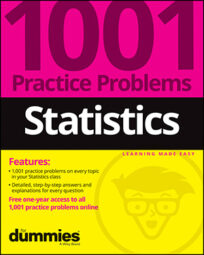##### Statistics: 1001 Practice Problems For Dummies (+ Free Online Practice)Be aware of the units of any descriptive statistic you calculate (for example, dollars, feet, or miles per gallon). Some descriptive statistics are in the same units as the data, and some aren't. Solve the following problems about data sets and descriptive statistics.

## Sample questions

1. Which of the following descriptive statistics is least affected by adding an outlier to a data set?

(A) the mean

(B) the median

(C) the range

(D) the standard deviation

(E) all of the above

The median of a data set is the middle value after you've put the data in order from smallest to largest (or the average of the two middle values if your data set contains an even number of values).

Because the median concerns only the very middle of the data set, adding an outlier won't affect its value much (if any). It adds only one more value to one end or the other of the sorted data set.

The mean is based on the sum of all the data values, which includes the outlier, so the mean will be affected by adding an outlier. The standard deviation involves the mean in its calculation; hence it's also affected by outliers.

The range is perhaps the most affected by an outlier, because it's the distance between the minimum and maximum values, so adding an outlier makes either the minimum value smaller or the maximum value larger. Either way, the distance between the minimum and maximum increases.

2. Which of the following statements is incorrect?

(A) The median and the 1st quartile can be the same.

(B) The maximum and minimum value can be the same.

(C) The 1st and 3rd quartiles can be the same.

(D) The range and the IQR can be the same.

(E) None of the above.

Answer: E. None of the above.

It's strange but true that all the scenarios are possible. You can use one data set as an example where all four scenarios occur at the same time: 5, 5, 5, 5, 5, 5, 5. In this case, the minimum and maximum are both 5, and the median (middle value) is 5. The median cuts the data set in half, creating an upper half and a lower half of the data set.

To find the 1st quartile, take the median of the lower half of the data set, which gives you 5 in this case; to find the 3rd quartile, take the median of the upper half of the data set (also 5). The range is the distance from the minimum to the maximum, which is 5 – 5 = 0.

The IQR is the distance from the 1st to the 3rd quartile, which is 5 – 5 = 0. Hence, the range and IQR are the same.

3. The average annual returns over the past ten years for 20 utility stocks have the following statistics:

1st quartile = 7

Median = 8

3rd quartile = 9

Mean = 8.5

Standard deviation = 2

Range = 5

Give the five numbers that make up the five-number summary for this data set.

Answer: The five-number summary can't be found.

The five-number summary of a data set includes the minimum value, the 1st quartile, the median, the 3rd quartile, and the maximum value. You're not given the minimum value or the maximum value here, so you can't fill out the five-number summary.

Note that even though you're given the range, which is the distance between the maximum and minimum values, you can't determine the actual values of the minimum and maximum.

4. Which of the following data sets has a mean of 15 and standard deviation of 0?

(A) 0, 15, 30

(B) 15, 15, 15

(C) 0, 0, 0

(D) There is no data set with a standard deviation of 0.

(E) Choices (B) and (C)

Many data sets containing three numbers can have a mean of 15. However, if you force the standard deviation to be 0, you have only one choice: 15, 15, 15. A standard deviation of 0 means the average distance from the data values to the mean is 0. In other words, the data values don't deviate from the mean at all, and hence they have to be the same value.

5. Which of the following statements is true?

(A) Fifty percent of the values in a data set lie between the 1st and 3rd quartiles.

(B) Fifty percent of the values in a data set lie between the median and the maximum value.

(C) Fifty percent of the values in a data set lie between the median and the minimum value.

(D) Fifty percent of the values in a data set lie at or below the median.

(E) All of the above.

Answer: E. All of the above.

A data set is divided into four parts, each containing 25% of the data: (1) the minimum value to the 1st quartile, (2) the 1st quartile to the median, (3) the median to the 3rd quartile, and (4) the 3rd quartile to the maximum value. Each statement represents a distance that covers two adjacent parts out of the four, which gives a total percentage of 25%(2) = 50% in every case.

If you need more practice on this and other topics from your statistics course, visit 1,001 Statistics Practice Problems For Dummies to purchase online access to 1,001 statistics practice problems! We can help you track your performance, see where you need to study, and create customized problem sets to master your stats skills.